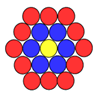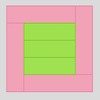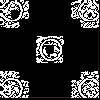#### You may also like### Counting Counters

Take a counter and surround it by a ring of other counters that MUST touch two others. How many are needed?### Cuisenaire Squares

These squares have been made from Cuisenaire rods. Can you describe the pattern? What would the next square look like?### Doplication

We can arrange dots in a similar way to the 5 on a dice and they usually sit quite well into a rectangular shape. How many altogether in this 3 by 5? What happens for other sizes?

# Button-up Some More

##### Age 7 to 11Challenge Level

Madeleine and Luke at Greystoke Primary worked carefully on four buttons:

We decided that we needed an organised way of working to make sure that we found all the different ways.
So we started with all the ways when you start with the first button, then all the ways you start with the second button etc.
In total we found $6$ ways for each so $6 \times 4 = 24$.
So we think that there are $24$ ways of buttoning up the coat.
Here are all the ways:
$1234$, $1243$, $1324$, $1342$, $1423$, $1432$, $2134$, $2143$, $2431$, $2413$, $2314$, $1341$, $3124$, $3142$, $3412$, $3421$, $3241$, $3214$, $4321$, $4312$, $4213$, $4231$, $4123$, $4132$
$6 \times 4 = 24$ ways.

Jordan from Birds Bush Primary gave some helpful detail about how we can make sure we don't miss any possibilities:

At the start of a solution start with the basic solution of '$1234$'.
Then switch the last two numbers,$1243$.
As you can't swap them again, swap the second number in the solution to another but not the first number.  In this case it's one eg: $1324$.
Now swap the last two numbers' places - $1342$.
As before you swap the second number in the solution with the only number which hasn't been second: $1432$.
And again swap the last two numbers' places - $1423$.
To get all solutions just use the same method but change the first number until all numbers have been in first place and you should end up with the $24$ different combinations as follows;
$1234$, $1243$, $1324$, $1342$, $1432$, $1423$, $2341$, $2314$, $2413$, $2431$, $2134$, $2143$, $3214$, $3241$, $3412$, $3421$, $3124$, $3142$, $4321$, $4312$, $4231$, $4213$, $4123$, $4132$.

Children from La Mare De Carteret Primary school agreed with 24 being the total number of ways for four buttons.

Scarlet, Will and Oliver, also from Greystoke Primary, investigate more buttons:

We looked at what happens when there are $5$ buttons on the coat.
Again we had an organised method and started by looking at all the ways when starting with the first button.
We found $24$ ways when starting with the first button, so we didn't need to do any more working, we just calculated $24 \times 5$ which is $120$ ways of buttoning up the coat with $5$ buttons.

I like the way you decided you didn't need to work out the number of ways for each button being first as it would be the same for all buttons.  Noticing short-cuts like that is a great skill for a mathematician.

Ella who goes to North Molton Primary looked at the number of ways to do up the buttons in a more general way.  Here is what she wrote:

First of all I got a piece of paper and drew all four buttons, naming them one, two, three and four.
Then, I experimented with the numbers, seeing how many different ways there were to order them.
I started of by working out how many different ways there were to do them up starting with button number one. There were six different ways. I did the same with buttons two, three and four. Each of them had six different ways.
So, all together there were twenty four different ways to do the four buttons up.
I tried the same method with three buttons. All together there were six different ways to do the three buttons up.
I realised that all the different ways for four buttons to be done up (twenty four) was the number of buttons (four) multiplied by the amount of ways a jacket could be buttoned up with one fewer button (six).
So, the way to work out how many ways there are to do up the jacket is the amount of buttons it has multiplied by the number of ways you can do it up with one less button.
For four buttons:
$4 \times 6 = 24$
(number of buttons) $\times$ (the number of ways to do it up with one less button) = amount of ways to do it up.

Very well noticed, Ella.  Dan and G from St Saviour's also suggested this was a good way to calculate the number of ways.

William from North Molton looked at it slightly differently.  He said:

I worked out that you have to times all the numbers you are using then you will get the answers e.g.
$1-4 = 1 \times 2 \times 3 \times 4 = 24$.
$1-5 = 1 \times 2 \times 3 \times 4 \times 5 = 120$.
$1-6 = 1 \times 2 \times 3 \times 4 \times 5 \times 6 = 720$.

Krystof from Uhelny Trh in Prague used a special symbol to write this down:

$2! = 2 \times1$
$3! = 3 \times 2 \times1$
$4! = 4 \times 3 \times 2 \times1$  etc.

Krystof explained, therefore, that for $n$ buttons there would be $n!$ different ways of buttoning them up.

Alex from Maidstone Grammar expressed the total number of ways of doing up a button slightly differently again:

There is a pattern to do with the factor of the numbers.
The first amount of sets is $6$, which equals $3 \times 2$.
The second set is a larger figure, $3 \times 8$.
Finally, $5$ buttons is a huge $3 \times 40$.
Each time, the second factor is multiplied larger.
It is first multiplied by $4$, to give $8$, and then $5$ to make $40$.
Therefore, $6$ buttons should equal $3 \times (40 \times 6)$ which is $3 \times 240$, giving $720$ combinations.

I wonder whether you can see how these different methods of expressing the total number of ways of doing up the buttons are connected?  If I used Alex's or Krystof's or William's or Ella's method, would I get the same answer, say for $10$ buttons?   Why?

Well done to you all.